# M.Ed Tcs 2 seminar ppt npc to submit

2. Nov 2021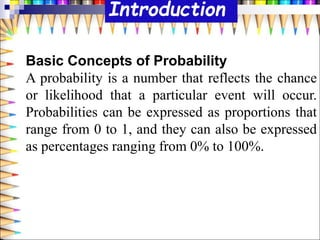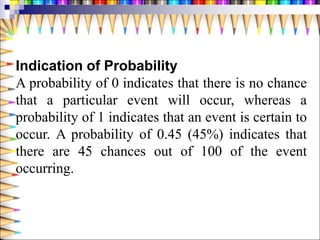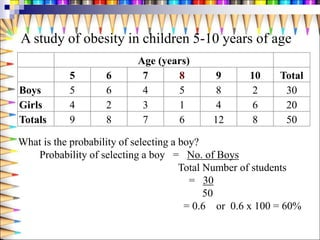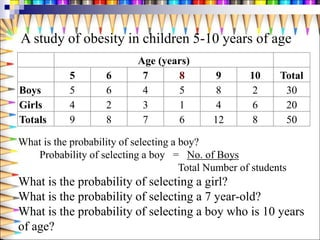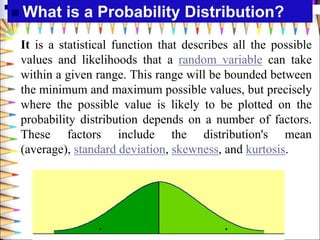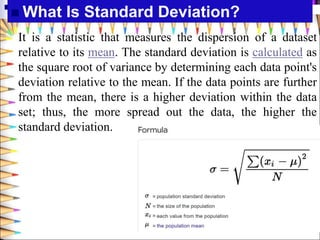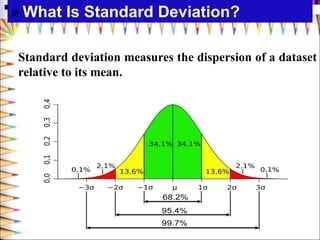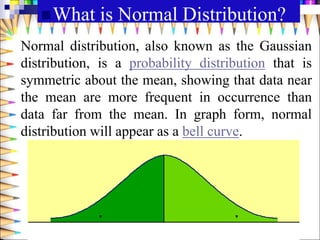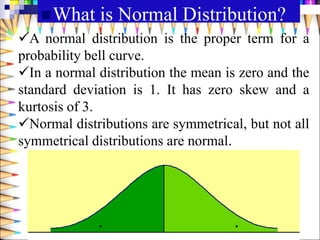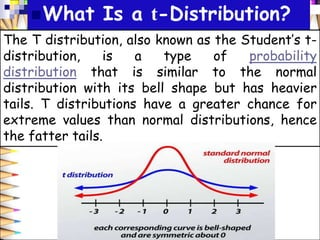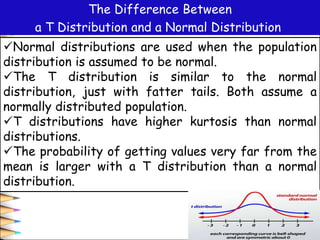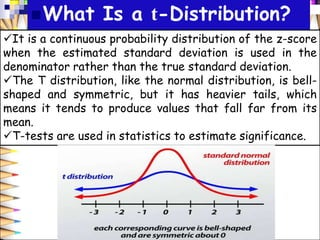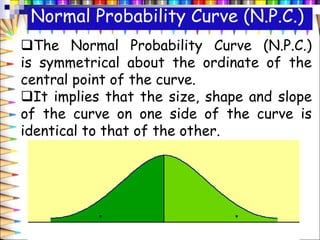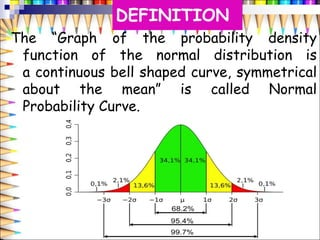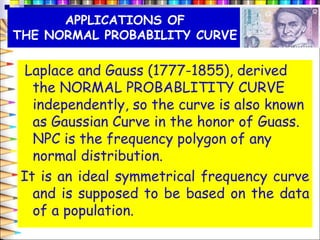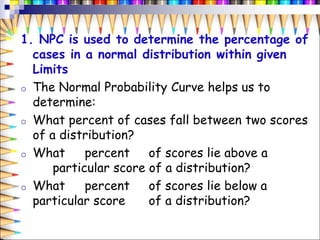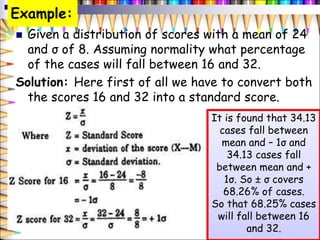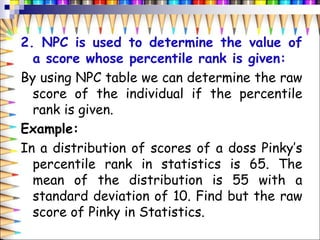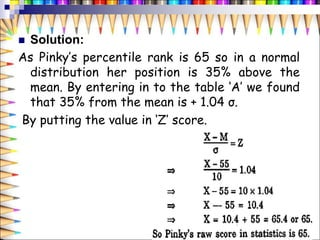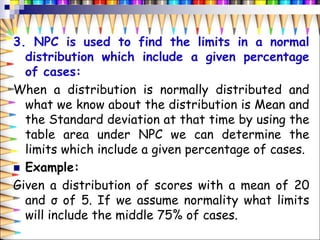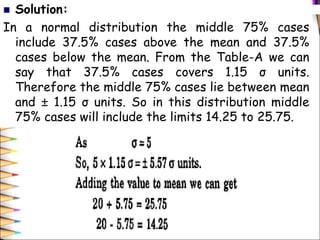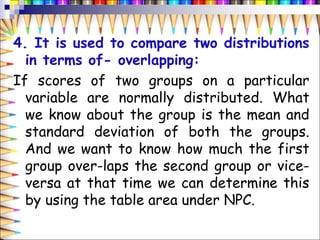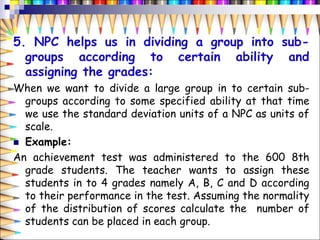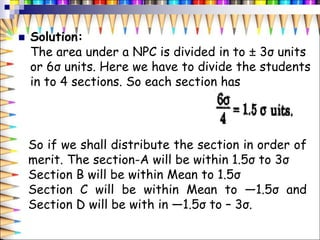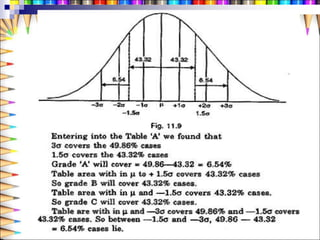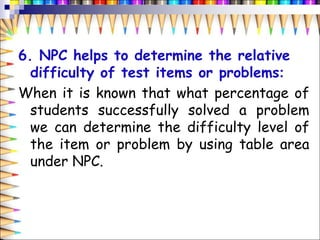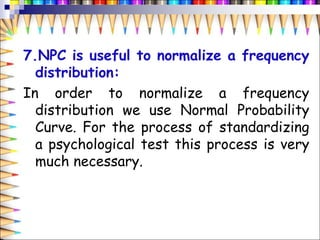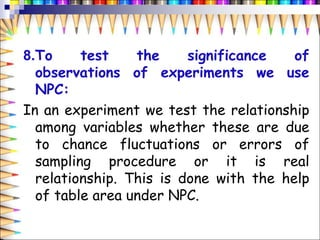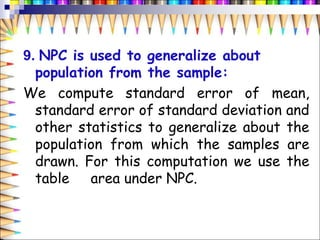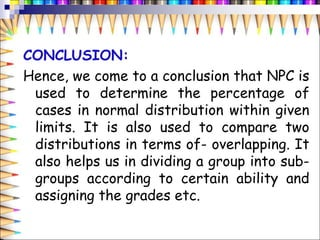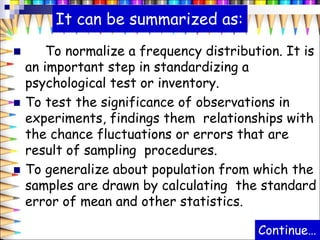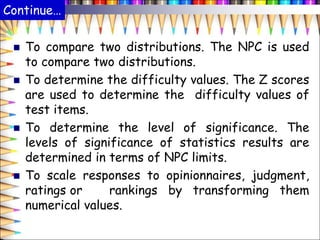1 von 34

### M.Ed Tcs 2 seminar ppt npc to submit

• 1. TCS 2: From Unit VI FUNDAMENTALS OF EDUCATIONAL REASERCH AND STATISTICS Prepared By Bincy K Mathew Reg. No.:20062
• 2. Introduction Basic Concepts of Probability A probability is a number that reflects the chance or likelihood that a particular event will occur. Probabilities can be expressed as proportions that range from 0 to 1, and they can also be expressed as percentages ranging from 0% to 100%.
• 3. Indication of Probability A probability of 0 indicates that there is no chance that a particular event will occur, whereas a probability of 1 indicates that an event is certain to occur. A probability of 0.45 (45%) indicates that there are 45 chances out of 100 of the event occurring.
• 4. A study of obesity in children 5-10 years of age Age (years) 5 6 7 8 9 10 Total Boys 5 6 4 5 8 2 30 Girls 4 2 3 1 4 6 20 Totals 9 8 7 6 12 8 50 What is the probability of selecting a boy? Probability of selecting a boy = No. of Boys Total Number of students = 30 50 = 0.6 or 0.6 x 100 = 60%
• 5. A study of obesity in children 5-10 years of age Age (years) 5 6 7 8 9 10 Total Boys 5 6 4 5 8 2 30 Girls 4 2 3 1 4 6 20 Totals 9 8 7 6 12 8 50 What is the probability of selecting a boy? Probability of selecting a boy = No. of Boys Total Number of students What is the probability of selecting a girl? What is the probability of selecting a 7 year-old? What is the probability of selecting a boy who is 10 years of age?
• 6.  What is a Probability Distribution? It is a statistical function that describes all the possible values and likelihoods that a random variable can take within a given range. This range will be bounded between the minimum and maximum possible values, but precisely where the possible value is likely to be plotted on the probability distribution depends on a number of factors. These factors include the distribution's mean (average), standard deviation, skewness, and kurtosis.
• 7.  What Is Standard Deviation? It is a statistic that measures the dispersion of a dataset relative to its mean. The standard deviation is calculated as the square root of variance by determining each data point's deviation relative to the mean. If the data points are further from the mean, there is a higher deviation within the data set; thus, the more spread out the data, the higher the standard deviation.
• 8.  What Is Standard Deviation? Standard deviation measures the dispersion of a dataset relative to its mean.
• 9. What is Normal Distribution? Normal distribution, also known as the Gaussian distribution, is a probability distribution that is symmetric about the mean, showing that data near the mean are more frequent in occurrence than data far from the mean. In graph form, normal distribution will appear as a bell curve.
• 10. What is Normal Distribution? A normal distribution is the proper term for a probability bell curve. In a normal distribution the mean is zero and the standard deviation is 1. It has zero skew and a kurtosis of 3. Normal distributions are symmetrical, but not all symmetrical distributions are normal.
• 11. What Is a t-Distribution? The T distribution, also known as the Student’s t- distribution, is a type of probability distribution that is similar to the normal distribution with its bell shape but has heavier tails. T distributions have a greater chance for extreme values than normal distributions, hence the fatter tails.
• 12. The Difference Between a T Distribution and a Normal Distribution Normal distributions are used when the population distribution is assumed to be normal. The T distribution is similar to the normal distribution, just with fatter tails. Both assume a normally distributed population. T distributions have higher kurtosis than normal distributions. The probability of getting values very far from the mean is larger with a T distribution than a normal distribution.
• 13. What Is a t-Distribution? It is a continuous probability distribution of the z-score when the estimated standard deviation is used in the denominator rather than the true standard deviation. The T distribution, like the normal distribution, is bell- shaped and symmetric, but it has heavier tails, which means it tends to produce values that fall far from its mean. T-tests are used in statistics to estimate significance.
• 14. Normal Probability Curve (N.P.C.) The Normal Probability Curve (N.P.C.) is symmetrical about the ordinate of the central point of the curve. It implies that the size, shape and slope of the curve on one side of the curve is identical to that of the other.
• 15. DEFINITION The “Graph of the probability density function of the normal distribution is a continuous bell shaped curve, symmetrical about the mean” is called Normal Probability Curve.
• 16. Laplace and Gauss (1777-1855), derived the NORMAL PROBABLITITY CURVE independently, so the curve is also known as Gaussian Curve in the honor of Guass. NPC is the frequency polygon of any normal distribution. It is an ideal symmetrical frequency curve and is supposed to be based on the data of a population. APPLICATIONS OF THE NORMAL PROBABILITY CURVE
• 17. 1. NPC is used to determine the percentage of cases in a normal distribution within given Limits o The Normal Probability Curve helps us to determine: o What percent of cases fall between two scores of a distribution? o What percent of scores lie above a particular score of a distribution? o What percent of scores lie below a particular score of a distribution?
• 18. Example:  Given a distribution of scores with a mean of 24 and σ of 8. Assuming normality what percentage of the cases will fall between 16 and 32. Solution: Here first of all we have to convert both the scores 16 and 32 into a standard score. It is found that 34.13 cases fall between mean and – 1σ and 34.13 cases fall between mean and + 1σ. So ± σ covers 68.26% of cases. So that 68.25% cases will fall between 16 and 32.
• 19. 2. NPC is used to determine the value of a score whose percentile rank is given: By using NPC table we can determine the raw score of the individual if the percentile rank is given. Example: In a distribution of scores of a doss Pinky’s percentile rank in statistics is 65. The mean of the distribution is 55 with a standard deviation of 10. Find but the raw score of Pinky in Statistics.
• 20.  Solution: As Pinky’s percentile rank is 65 so in a normal distribution her position is 35% above the mean. By entering in to the table ‘A’ we found that 35% from the mean is + 1.04 σ. By putting the value in ‘Z’ score.
• 21. 3. NPC is used to find the limits in a normal distribution which include a given percentage of cases: When a distribution is normally distributed and what we know about the distribution is Mean and the Standard deviation at that time by using the table area under NPC we can determine the limits which include a given percentage of cases.  Example: Given a distribution of scores with a mean of 20 and σ of 5. If we assume normality what limits will include the middle 75% of cases.
• 22.  Solution: In a normal distribution the middle 75% cases include 37.5% cases above the mean and 37.5% cases below the mean. From the Table-A we can say that 37.5% cases covers 1.15 σ units. Therefore the middle 75% cases lie between mean and ± 1.15 σ units. So in this distribution middle 75% cases will include the limits 14.25 to 25.75.
• 23. 4. It is used to compare two distributions in terms of- overlapping: If scores of two groups on a particular variable are normally distributed. What we know about the group is the mean and standard deviation of both the groups. And we want to know how much the first group over-laps the second group or vice- versa at that time we can determine this by using the table area under NPC.
• 24. 5. NPC helps us in dividing a group into sub- groups according to certain ability and assigning the grades: When we want to divide a large group in to certain sub- groups according to some specified ability at that time we use the standard deviation units of a NPC as units of scale.  Example: An achievement test was administered to the 600 8th grade students. The teacher wants to assign these students in to 4 grades namely A, B, C and D according to their performance in the test. Assuming the normality of the distribution of scores calculate the number of students can be placed in each group.
• 25.  Solution: The area under a NPC is divided in to ± 3σ units or 6σ units. Here we have to divide the students in to 4 sections. So each section has So if we shall distribute the section in order of merit. The section-A will be within 1.5σ to 3σ Section B will be within Mean to 1.5σ Section C will be within Mean to —1.5σ and Section D will be with in —1.5σ to – 3σ.
• 27. 6. NPC helps to determine the relative difficulty of test items or problems: When it is known that what percentage of students successfully solved a problem we can determine the difficulty level of the item or problem by using table area under NPC.
• 28. 7.NPC is useful to normalize a frequency distribution: In order to normalize a frequency distribution we use Normal Probability Curve. For the process of standardizing a psychological test this process is very much necessary.
• 29. 8.To test the significance of observations of experiments we use NPC: In an experiment we test the relationship among variables whether these are due to chance fluctuations or errors of sampling procedure or it is real relationship. This is done with the help of table area under NPC.
• 30. 9. NPC is used to generalize about population from the sample: We compute standard error of mean, standard error of standard deviation and other statistics to generalize about the population from which the samples are drawn. For this computation we use the table area under NPC.
• 31. CONCLUSION: Hence, we come to a conclusion that NPC is used to determine the percentage of cases in normal distribution within given limits. It is also used to compare two distributions in terms of- overlapping. It also helps us in dividing a group into sub- groups according to certain ability and assigning the grades etc.
• 32. It can be summarized as:  To normalize a frequency distribution. It is an important step in standardizing a psychological test or inventory.  To test the significance of observations in experiments, findings them relationships with the chance fluctuations or errors that are result of sampling procedures.  To generalize about population from which the samples are drawn by calculating the standard error of mean and other statistics. Continue…
• 33. Continue…  To compare two distributions. The NPC is used to compare two distributions.  To determine the difficulty values. The Z scores are used to determine the difficulty values of test items.  To determine the level of significance. The levels of significance of statistics results are determined in terms of NPC limits.  To scale responses to opinionnaires, judgment, ratings or rankings by transforming them numerical values.